# What Is Triode Region in MOSFET?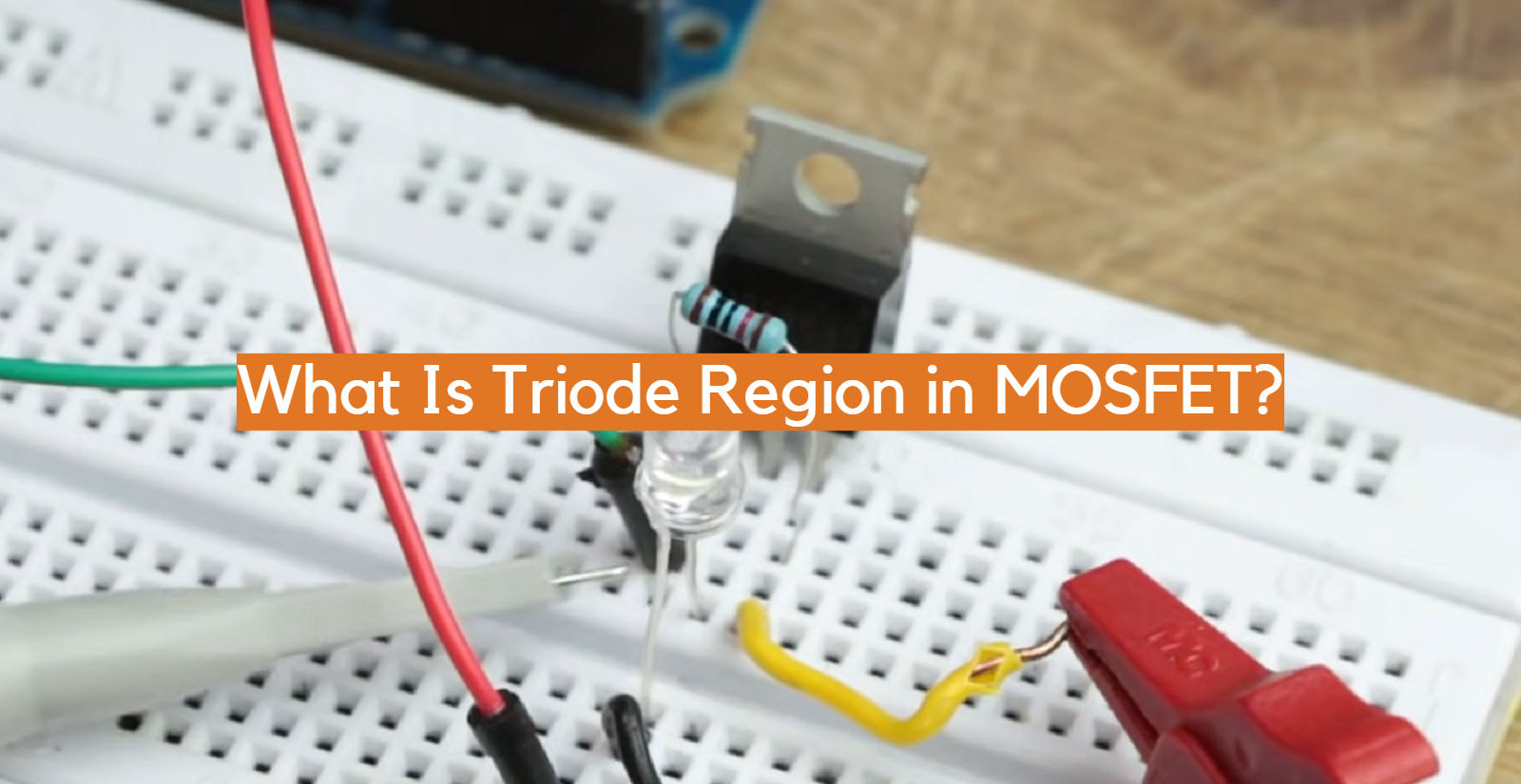The triode region is a critical operating region of the Metal-Oxide-Semiconductor Field-Effect Transistor (MOSFET), an essential electronic device with versatile applications. In the triode region, the MOSFET acts as a voltage-controlled current amplifier, allowing precise control over the current flowing between its source and drain terminals. This region is characterized by a linear relationship between the gate-source voltage and the drain current, making it suitable for various analog applications that require amplification and signal processing.

Understanding the triode region is crucial for engineers and electronics enthusiasts as it unlocks the potential of the MOSFET in designing efficient and high-performance circuits. In this article, we will delve into the intricacies of the triode region and explore its significance in MOSFET operation.

## What Is MOSFET?

MOSFET, short for Metal-Oxide-Semiconductor Field-Effect Transistor, is a fundamental electronic device that plays a crucial role in modern technology. It is a type of field-effect transistor (FET) that is widely used in a variety of electronic circuits due to its ability to efficiently switch and amplify electrical signals .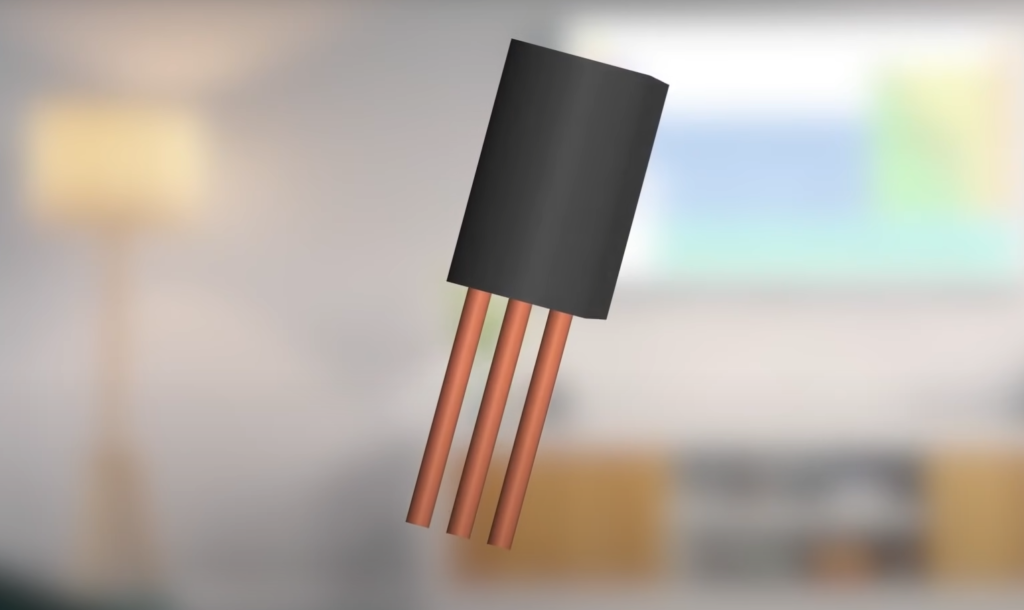The basic structure of a MOSFET consists of three main components: a semiconductor substrate, a metal gate, and a thin layer of insulating material known as the oxide layer. The semiconductor substrate is typically made of silicon, and it acts as the channel through which current flows. The oxide layer, usually made of silicon dioxide (SiO2), separates the gate from the channel.

The operation of a MOSFET relies on the electric field created by the voltage applied to the gate terminal. There are two main types of MOSFETs: the enhancement mode and the depletion mode:

• In an enhancement-mode MOSFET, the device is normally off when no voltage is applied to the gate. However, when a positive voltage is applied, it creates an electric field that attracts free charge carriers, allowing current to flow between the source and the drain terminals;
• In a depletion-mode MOSFET, the device is normally on, and applying a negative voltage to the gate turns it off by depleting the channel of charge carriers;

One of the key advantages of MOSFETs is their high input impedance, which means they have a minimal effect on the signal source they are connected to. This makes them well-suited for use in high-gain amplifier circuits. Additionally, MOSFETs have low power consumption, fast switching speeds, and high voltage-handling capabilities. These characteristics make them ideal for various applications, including power amplifiers, digital logic circuits, voltage regulators, and switching regulators.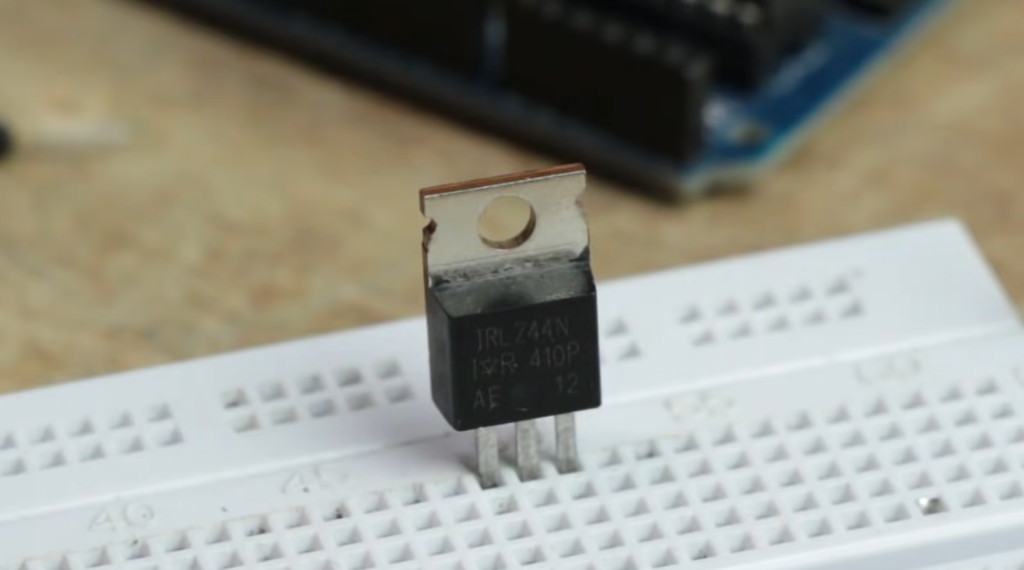Furthermore, MOSFETs have significantly contributed to the miniaturization of electronic devices. With advancements in semiconductor fabrication technology, MOSFETs can now be manufactured on a microscopic scale, allowing for the integration of millions or even billions of transistors on a single integrated circuit (IC) chip. This has led to the development of powerful microprocessors, memory chips, and other complex digital circuits that have revolutionized industries such as computing, telecommunications, and consumer electronics.

## 3 Regions Of Operation Of A MOSFET:

### 1) Cut-Off Region

The Cut-Off region, also known as the off state, is the region where the MOSFET is effectively turned off, and no current flows between the source and the drain terminals. In this state, the voltage applied to the gate terminal is below the threshold voltage (Vth) of the MOSFET.

The threshold voltage is the minimum voltage required to create a conducting channel between the source and the drain.
When the gate voltage is below the threshold voltage, the MOSFET’s channel is depleted of charge carriers, preventing the flow of current.

The MOSFET behaves as an open circuit in this region, effectively blocking the passage of electrical signals. The Cut-Off region is crucial for ensuring that the MOSFET does not consume unnecessary power when it is not actively being used.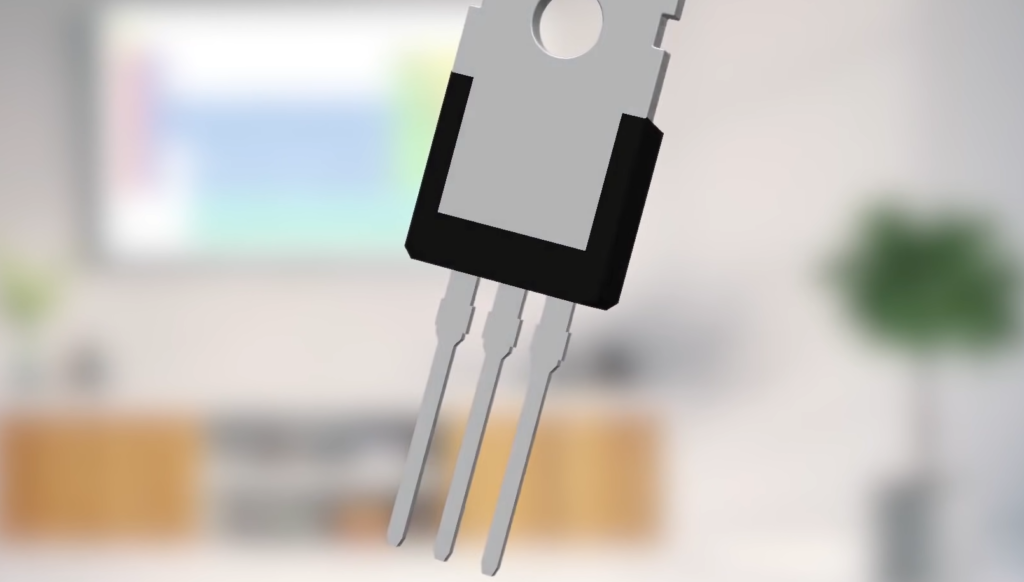### 2) Triode Region

The Triode region, also known as the linear region or the active region, is the region where the MOSFET operates as a voltage-controlled current amplifier. In this region, the gate voltage exceeds the threshold voltage, allowing a conducting channel to form between the source and the drain terminals.

When a small positive voltage is applied to the gate terminal, it creates an electric field that attracts charge carriers, enabling current flow through the channel. The amount of current flowing in the Triode region is directly proportional to the gate voltage. The MOSFET operates as a variable resistor, with the resistance determined by the gate-source voltage (Vgs).

In the Triode region, the MOSFET behaves as a voltage-controlled switch or an amplifier. It is commonly used in analog circuits, such as amplifiers and signal processing circuits, where precise control over the current is required.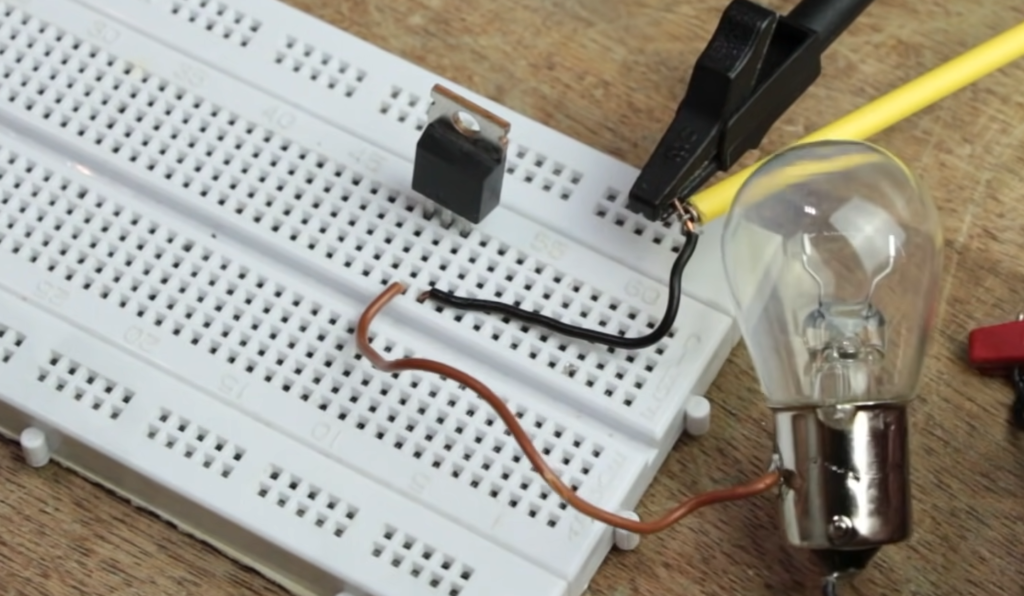### 3) Saturation Region

The Saturation region, also known as the on state, is the region where the MOSFET operates as a closed switch, allowing maximum current flow between the source and the drain terminals. In this region, the gate voltage is significantly higher than the threshold voltage, creating a fully conducting channel.

When a sufficiently high positive voltage is applied to the gate terminal, it attracts a large number of charge carriers, resulting in a low resistance path between the source and the drain. The MOSFET operates as a closed switch, allowing current to flow freely.

The Saturation region is commonly used in digital circuits, such as logic gates and memory cells. In these applications, the MOSFET is used as a switch to control the flow of binary signals (0s and 1s). The low resistance in the Saturation region ensures a strong and reliable current flow, enabling efficient digital signal processing .

## What Is Triode Region In MOSFET?

The triode region is a part of the linear or resistive region of MOSFET.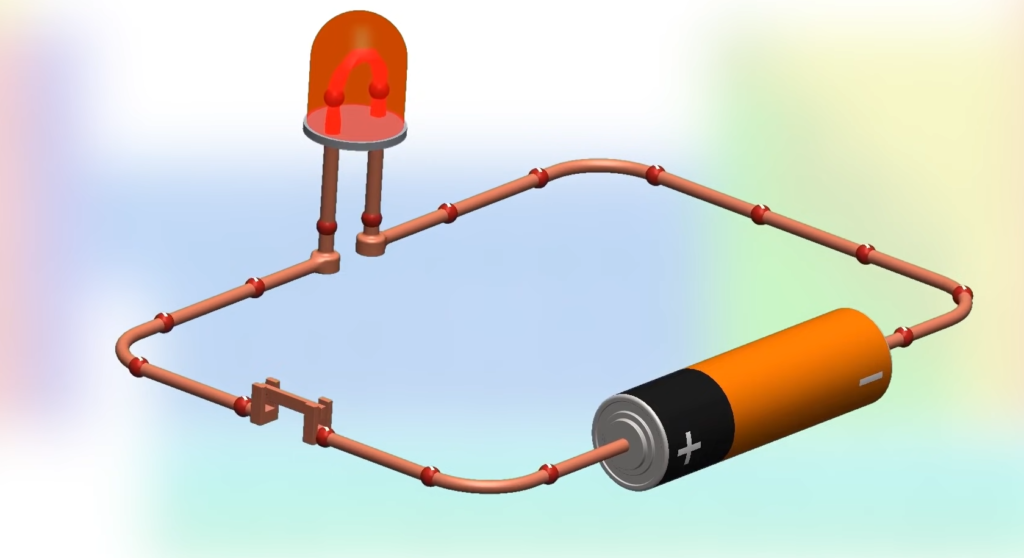In this region, the MOSFET operates like a triode vacuum tube. In triode mode, the MOSFET is used as a voltage-controlled resistor, which means the resistance between the drain and source is controlled by the gate voltage. In the triode region, the MOSFET behaves like a voltage-controlled current source, and the output current is proportional to the input voltage applied to the gate.

The I-V characteristics of a MOSFET consist of the drain current (I_D) on the Y-axis and the drain-source voltage (V_DS) on the X-axis. The drain current and the drain-source voltage are related to the gate-source voltage (V_GS) applied to the MOSFET.

In the cutoff region, the MOSFET is off, and there is no current flow through the device. In this region, the gate-source voltage is less than the threshold voltage (V_TH), which is the minimum voltage required to turn the MOSFET on. When the gate-source voltage is greater than V_TH, the MOSFET enters the linear or resistive region.

In the linear or resistive region, the MOSFET operates like a variable resistor, and the drain current is proportional to the gate-source voltage. As we increase the gate-source voltage, the MOSFET resistance decreases, and the drain current increases linearly. In the linear region, the MOSFET can be used for the amplification of small signals.

In the triode region, the MOSFET operates like a triode vacuum tube, and the drain current is no longer proportional to the gate-source voltage, but it follows the triode equation. The triode equation relates the grid voltage, the plate current, and the cathode-resistor bias voltage. The triode region is typically used for audio applications, where the MOSFET is used as a voltage-controlled resistor for audio amplification.

## Why Does a Triode Region Matter?

The triode region in a MOSFET is a region of operation where the MOSFET behaves like a voltage-controlled resistor, like a triode vacuum tube. It is part of the linear or resistive region of the MOSFET, and it is different from both the cutoff and saturation regions of operation.

The purpose of using the triode region in a MOSFET is to exploit its voltage-controlled resistance properties for audio amplification and other applications that require voltage-controlled resistance.

The MOSFET is a versatile device that can be used for switching, amplification, and voltage regulation, among other applications . By utilizing the triode region of operation, the MOSFET can provide a linear response to small signals, making it suitable for audio amplification. The triode region can be identified by the presence of an inversion layer between the source and drain, and the drain current is proportional to the gate-source voltage.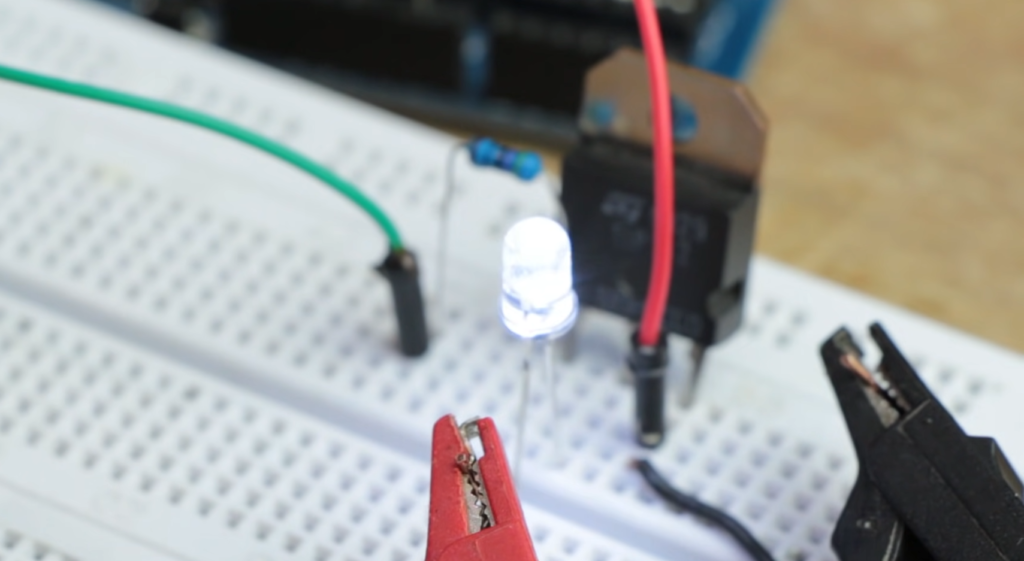This region can be useful for circuit designers who want to exploit the MOSFET’s electronic properties to achieve a specific goal, such as amplifying an audio signal or controlling a voltage.

## What Is The Difference Between A Triode And A Transistor?

A triode and a transistor are both electronic devices used for amplification and switching purposes, but they are fundamentally different in their construction and operation.

A triode is a vacuum tube electronic amplifying device consisting of three electrodes – a heated cathode, a control grid, and a plate (or anode) – inside an evacuated glass envelope. It amplifies a small signal by controlling the current flow through the tube by varying the voltage applied to the control grid. The triode was widely used in early electronic equipment, such as radios and televisions, but has been largely supplanted by the transistor in modern electronics.

A transistor, on the other hand, is a semiconductor device consisting of three layers of doped materials – a p-type layer sandwiched between two n-type layers (PNP transistor) or an n-type layer sandwiched between two p-type layers (NPN transistor) – that act as either a switch or an amplifier.

The transistor is controlled by applying a voltage to the base (for a bipolar transistor) or the gate (for a field-effect transistor), which allows or prevents current to flow between the collector (or drain) and emitter (or source).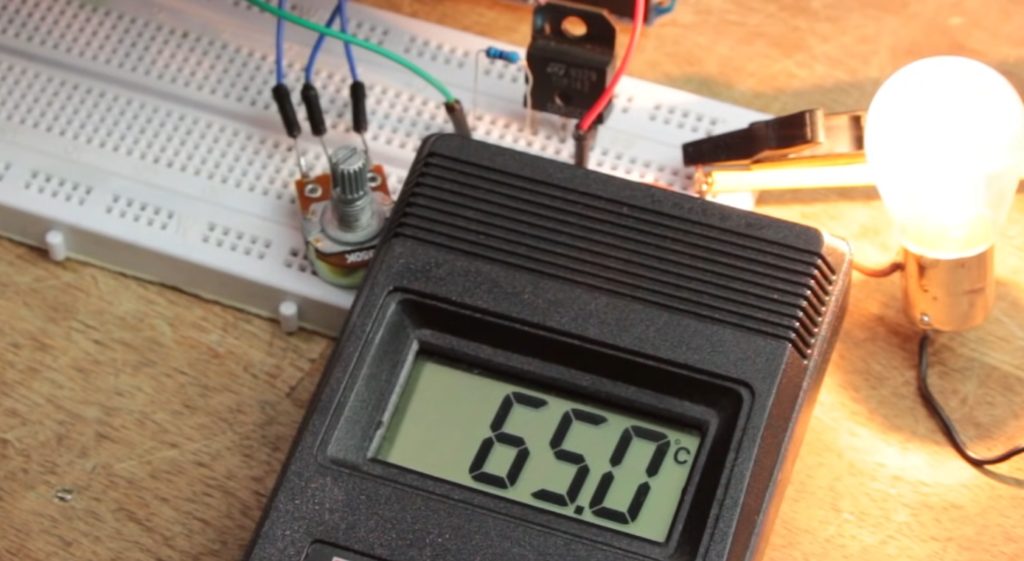## FAQ:

### 1. What is the most common triode?

The 12A*7 family, including the 12AT7 and 12AX7 high-dual triodes, are the most popular of the triodes. The 12AU7 and 12AY7 medium gain dual triodes are also widely used . However, it’s worth noting that the most common triode can vary depending on the specific application and the preferences of the circuit designer.

### 2. Is the triode region the same as linear?

Yes, the triode region is often referred to as the linear region. In this region, the MOSFET operates as a voltage-controlled current amplifier, and the drain current is directly proportional to the gate-source voltage.

### 3. How do I know if my MOSFET is in saturation or triode?

To determine whether a MOSFET is in saturation or triode, you need to compare the gate-source voltage (Vgs) with the threshold voltage (Vth) of the MOSFET. If Vgs is significantly higher than Vth, the MOSFET is in saturation. If Vgs is lower than Vth, the MOSFET is in the triode or linear region.

### 4. Why is the MOSFET in the saturation region?

The MOSFET is in the saturation region when a sufficiently high positive voltage is applied to the gate terminal. In this region, the MOSFET acts as a closed switch, allowing maximum current flow between the source and the drain. Saturation is desired in applications where the MOSFET needs to provide a strong and reliable current flow, such as in digital circuits.

### 5. Why is the triode important?

The triode region of a MOSFET is important because it allows the device to function as a voltage-controlled current amplifier. This characteristic makes it suitable for various analog applications where precise control over the current is required, such as in amplifiers, signal processing circuits, and other analog circuits.

### 6. Is the triode passive or active?

The triode region is considered an active region. In this region, the MOSFET actively controls the flow of current between the source and the drain terminals based on the voltage applied to the gate terminal.

### 7. Is the triode region the Ohmic region?

No, the triode region is not the Ohmic region. The Ohmic region refers to the region of operation of a device where the current is directly proportional to the applied voltage, obeying Ohm’s law. The triode region is characterized by a voltage-controlled current, not a linear relationship like Ohm’s law .

### 8. What is a triode and its characteristics?

A triode is a type of vacuum tube that consists of three elements: a cathode, an anode (plate), and a control grid. It is used for signal amplification and switching. The key characteristics of a triode include its ability to amplify weak electrical signals, its high input impedance, and the ability to control the electron flow through the control grid.

### 9. Is a transistor a triode?

No, a transistor is not a triode. While a triode is a specific type of vacuum tube, a transistor is a solid-state electronic device that amplifies or switches electronic signals. Transistors can be classified into different types such as bipolar junction transistors (BJTs) and field-effect transistors (FETs), including MOSFETs.

### 10. Is a triode a rectifier?

No, a triode is not a rectifier. A rectifier is a device used to convert alternating current (AC) into direct current (DC) by allowing current to flow in only one direction. A triode, on the other hand, is primarily used for amplification and signal processing.

### 11. What is the difference between a diode and a triode?

A diode is a two-terminal electronic device that allows current to flow in only one direction. It acts as a switch for electrical signals. A triode, on the other hand, is a three-element vacuum tube or a specific configuration of a transistor that is used for signal amplification and switching. While both diodes and triodes control the flow of current, their operating principles and applications differ.

### 12. How does a triode amplify a signal?

A triode amplifies a signal through the control of electron flow using the control grid. By applying a varying voltage to the control grid, the triode modulates the flow of electrons from the cathode to the anode (plate), thus amplifying the input signal. The amplification is achieved by controlling the number of electrons passing through the control grid, resulting in a larger output signal.

### 13. Is a TRIAC a triode?

No, a TRIAC is not a triode. A TRIAC is a semiconductor device that is used to control alternating current (AC) power. It is commonly used in applications such as dimmer switches and motor speed control. While the name TRIAC contains “tri” as a prefix, it does not refer to a triode but rather signifies its ability to control current in both directions.

### 14. Is a triode a resistor?

No, a triode is not a resistor. A triode is an active electronic device that controls the flow of current through the modulation of electron flow using the control grid. A resistor, on the other hand, is a passive electronic component that opposes the flow of current and dissipates electrical energy in the form of heat.

### 15. What are the limitations of a triode?

Some limitations of a triode include limitations in high-frequency response, limited power handling capabilities, and the requirement of high voltage for operation. Additionally, triodes are bulky, require high-voltage power supplies, and are sensitive to temperature variations. These limitations led to the development of more advanced electronic devices like transistors and integrated circuits.

### 16. What is the condition for the triode region?

The condition for the MOSFET to operate in the triode region is that the gate-source voltage (Vgs) must be higher than the threshold voltage (Vth). In this region, the MOSFET functions as a voltage-controlled current amplifier, allowing precise control over the current flow between the source and the drain terminals.

### 17. Which transistor is equivalent to a triode?

A bipolar junction transistor (BJT) can be considered as an equivalent to a triode in terms of its amplification characteristics. Both devices are used for signal amplification, and while their structures and operating principles differ, they share similarities in amplifying weak signals.

### 18. Does a MOSFET have a diode?

Yes, a MOSFET has an inherent diode known as the body diode. This diode is formed by the PN junction between the source and the drain regions in the MOSFET structure. The body diode conducts current in the reverse direction when a voltage is applied across the drain and source terminals with the gate terminal at a lower voltage.

### 19. What is the Ohmic region?

The Ohmic region, also known as the linear region, refers to the region of operation of a device where the current is directly proportional to the applied voltage, following Ohm’s law. In this region, the device behaves as a linear resistor, allowing easy control of the current flow.

### 20. What are the three parameters of a triode?

The three key parameters of a triode are the amplification factor (µ), transconductance (gm), and plate resistance (rp). The amplification factor represents the gain of the triode, transconductance measures the change in plate current with respect to the change in grid voltage, and plate resistance represents the dynamic resistance of the triode.

### 21. Is a MOSFET active or passive?

A MOSFET (Metal-Oxide-Semiconductor Field-Effect Transistor) is an active electronic device. It actively controls the flow of current based on the voltage applied to the gate terminal. By modulating the electric field in the channel, the MOSFET can amplify signals and act as a switch.

### 22. What is a triode connection?

The term “triode connection” can refer to a specific configuration or wiring arrangement of electronic components, particularly vacuum tubes or transistors. In the case of vacuum tubes, a triode connection involves the connection of the cathode, grid, and plate in a specific manner to enable amplification or signal processing. For transistors, the term may refer to the configuration of the terminals for a specific application, such as a common-emitter configuration in a BJT transistor.

### 23. Is the MOSFET switch linear or saturation?

The MOSFET can operate in different regions depending on the voltage applied to its terminals. When the MOSFET is in saturation, it operates as a closed switch, allowing maximum current flow between the source and the drain. When it is in the linear region, also known as the triode region, it functions as a voltage-controlled current amplifier. So, the MOSFET can be used as both a switch (saturation) and an amplifier (linear/triode).

### 24. What is the MOSFET threshold region?

The MOSFET threshold region is the region of operation where the gate voltage is slightly higher than the threshold voltage (Vth) but not high enough to fully turn on the MOSFET. In this region, the MOSFET is transitioning between the cut-off region and the saturation region. The behavior of the MOSFET in the threshold region is nonlinear and is typically avoided in most applications.

### 25. What is constant K in MOSFET?

In the context of MOSFETs, the constant K refers to the transconductance parameter or the amplification factor . It represents the ratio of the change in drain current to the change in gate-source voltage. The transconductance parameter is denoted by the symbol “k” or “μ”, and it quantifies the amplification capability of the MOSFET.

### 26. What are the four types of MOSFET?

The four main types of MOSFETs are:

• Enhancement-mode N-channel MOSFET (NMOS): The channel is enhanced when a positive voltage is applied to the gate;
• Enhancement-mode P-channel MOSFET (PMOS): The channel is enhanced when a negative voltage is applied to the gate;
• Depletion-mode N-channel MOSFET (DNMOS): The channel is depleted when a negative voltage is applied to the gate;
• Depletion-mode P-channel MOSFET (DPMOS): The channel is depleted when a positive voltage is applied to the gate;